# Macroeconomics Study Set 62

## Quiz 10 : Bringing in the Supply Side: Unemployment and InflationStudy FlashcardsLooking for Economics Homework Help?

## Quiz 10 :Bringing in the Supply Side: Unemployment and Inflation

Question TypeGive two different explanations of how the economy can suffer from stagflation.
Free
Essay

Stagflation refers to the state where the economy has slow growth rate accompanied by higher unemployment rate and high price level. It means unemployment rate and inflation rate move together.
The impact of stagflation on the economy
Stagflation itself results in a slow growth process, and the economists distinguish two different effects by stagflation.
• Stagflation is the situation where the aggregate demand is above the employment level. This results in an inflationary gap in the economy; when stagflation arises at this stage, it makes the economy to prolong with the same condition for longer periods of time.
• The combination of stagnation and inflation affects the economy with an increase in prices for a long period. This slows down the economic process.
• At the initial level, the prices and output rise; later, the costs chase the prices. This decreases the aggregate supply. Here, the stagflation is obvious; where the rise in price and fall in the output exist.
• There is no tradeoff between inflation and unemployment ; the state of stagflation increases unemployment, and there would be a constant wage system, which worsens the economy.
Hence, the two factors stated above hook up the economy in a phase where there will be a slow economic process. These are the phenomena the economy suffers from stagflation.

Tags
Choose question tagUse an aggregate supply-and-demand diagram to show that multiplier effects are smaller when the aggregate supply curve is steeper. Which case gives rise to more inflation-the steep aggregate supply curve or the flat one What happens to the multiplier if the aggregate supply curve is vertical
Free
Essay

Steep Aggregate Supply
When the aggregate supply curve is steeper, the multiplier effect of an increase in aggregate demand is smaller because it increases the level of output by a smaller amount.
Graphical Representation
The following diagram shows the impact of steeper aggregate supply curve.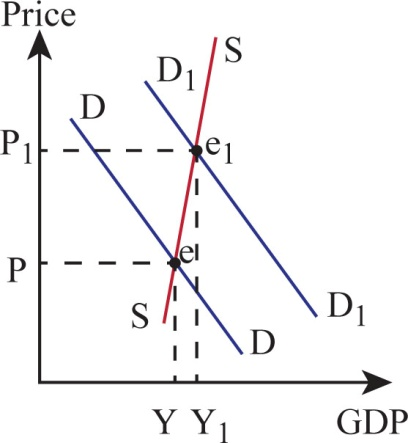Diagram (1)
In the above diagram, horizontal axis represents the gross domestic product (GDP) and vertical axis represents the price level. The blue line represents the demand curve and red line represents the supply curve.
The initial demand and supply curves are D and S, respectively, and the intersection of the two curves determines the equilibrium level of price and output. When there is an increase in the aggregate demand, the demand curve shifts rightward from D to D₁. The new demand curve intersects the supply curve at e₁ , the equilibrium level of output increases from Y to Y₁ , and price level increases from P to P₁. Hence, the output increases by a smaller amount.
Flatter Aggregate Supply
When the aggregate supply curve is flatter, there is an equal increase in aggregate demand, which increases the level of output by a larger amount.
Graphical Representation
The following diagram shows the impact of flatter aggregate supply curve.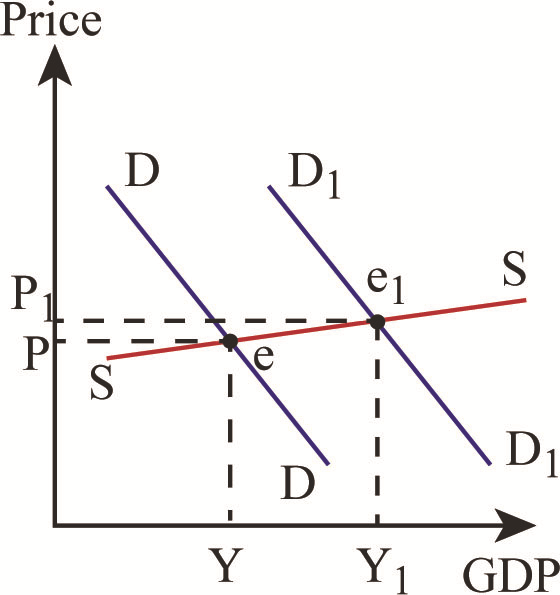Diagram (2)
In the above diagram, horizontal axis represents the gross domestic product (GDP) and vertical axis represents the price level. The blue line represents the demand curve and red line represents the supply curve.
The initial demand and supply curve are D and S, respectively, and the intersection of the two curves determines the equilibrium level of price and output. When there is an increase in the aggregate demand, the demand curve shifts rightward from D to D₁. The new demand curve intersects the supply curve at e₁ , the equilibrium level of output increases from Y to Y₁ , and price level increases from P to P₁. Hence, the above diagram clearly shows that the output increases by a larger amount.
Vertical Aggregate Supply Curve
When the aggregate supply curve is vertical and aggregate demand curve increases, this leads to an increase in the general price level, thereby resulting in inflation. The impact of multiplier is zero.
Graphical Representation
The following diagram shows the impact of vertical aggregate supply curve.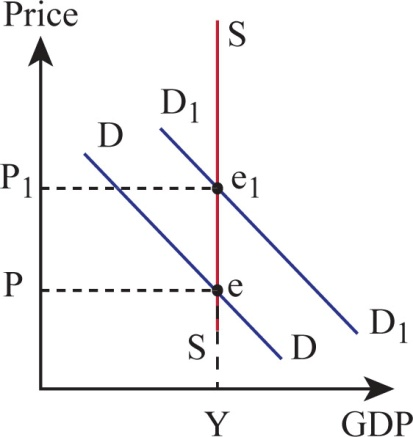Diagram (3)
In the above diagram, horizontal axis represents the gross domestic product (GDP) and vertical axis represents the price level. The blue line represents the demand curve and red line represents the supply curve.
The initial demand and supply curve are D and S, respectively, and the intersection of the two curves determines the equilibrium level of price and output. When there is an increase in the aggregate demand, the demand curve shifts rightward from D to D₁. The new demand curve intersects the supply curve at e₁ , and the equilibrium level of output does not change from Y₁. The above diagram clearly shows that there is no change in the level of output, but price level increases from P to P₁ when there is an increase in the aggregate demand.

Tags
Choose question tagIn an economy with the following aggregate demand and aggregate supply schedules, find the equilibrium levels of real output and the price level. Graph your solution. If full employment comes at $2,800 billion, is there an inflationary or a recessionary gap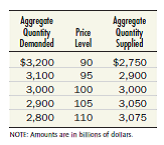Free Essay Answer: Answer: Recessionary Gap Recessionary gap arises when aggregate demand is lower than the aggregate supply and short run equilibrium in the economy is below the full employment level. Graphical Representation The following figure shows whether the economy has inflationary or recessionary gap.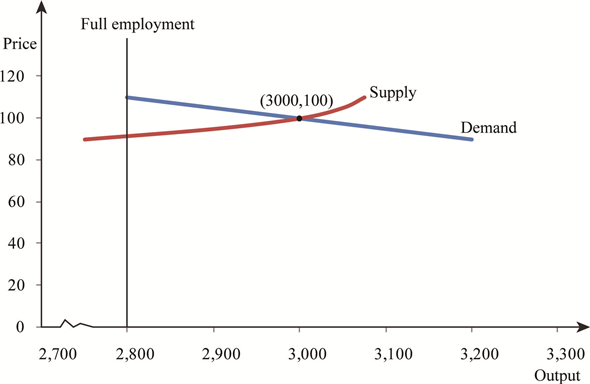Figure-2 In the above figure, X axis represents the different levels of output and Y axis represents the different levels of price. Here, the upward sloping curve is a supply curve and downward sloping curve is a demand curve. Figure (2) clearly indicates that there exists an inflationary gap when the full employment level of output is$2,800. This is because the equilibrium level of output $3,000 is greater than the full employment level of output$2,800.

Tags
Choose question tagWhy do you think wages tend to be rigid in the downward direction
Essay
Tags
Choose question tagComment on the following statement: "Inflationary and recessionary gaps are nothing to worry about because the economy has a built-in mechanism that cures either type of gap automatically."
Essay
Tags
Choose question tagAdd the aggregate supply and demand schedules below to the example in Test Yourself Question 1 of Chapter 9 (page₁92) to see how inflation affects the multiplier.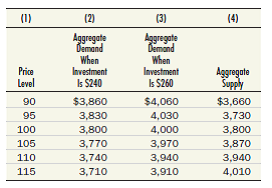Draw these schedules on a piece of graph paper. a. Notice that the difference between columns (2) and (3), which show the aggregate demand schedule at two different levels of investment, is always $200. Discuss how this constant gap of$200 relates to your answer in the previous chapter. b. Find the equilibrium GDP and the equilibrium price level both before and after the increase in investment. What is the value of the multiplier Compare that to the multiplier you found in Test Yourself Question 2 of Chapter 9. Reference Question 1 of Chapter 9 Suppose exports and imports of a country are given by the following: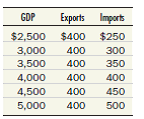Reference Question 2 of Chapter 9 If domestic expenditure (the sum of C + I + G in the economy described in Test Yourself Question 1) is as shown in the following table, construct a 45° line diagram and locate the equilibrium level of GDP.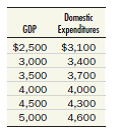Essay
Tags
Choose question tagExplain in words why rising prices reduce the multiplier effect of an autonomous increase in aggregate demand.
Essay
Tags
Choose question tagExplain why a decrease in the price of foreign oil shifts the aggregate supply curve outward to the right. What are the consequences of such a shift
Essay